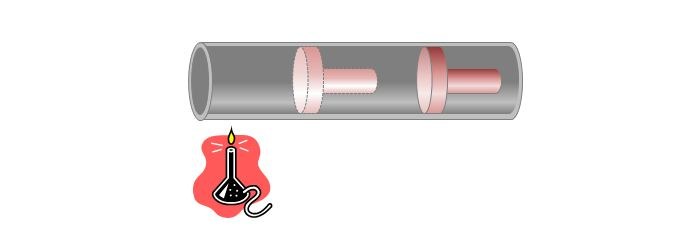# Heating Ideal gasThe above picture shows a monoatomic ideal gas in a cylinder being heated under the constant pressure of $1\times 10^5$ Pa. At the initial state before heating, the temperature and volume of the ideal gas were $300$ K and $1\times 10^{-3} \text{ m}^3$, respectively. After heating, its volume has become $3 \times 10^{-3} \text{ m}^3.$ Which of the following statements is correct?

a) The temperature of the ideal gas after heating is $600$ K.

b) The work done by the ideal gas is $200$ J.

c) The heat absorbed by the ideal gas is $500$ J.

×

Problem Loading...

Note Loading...

Set Loading...